## Lindsey Wilson was the top scorer in a women’s professional basketball league for the 2006 regular season, with a total of 814 points. The n

Question

Lindsey Wilson was the top scorer in a women’s professional basketball league for the 2006 regular season, with a total of 814 points. The number of two-point field

goals that Lindsey made was 49 less than double the number of three-point field goals she made. The number of free throws (each worth one point) she made was

38 less than the number of two-point field goals she made. Find how many free throws, two-point field goals, and three-point field goals Lindsey Wilson made during

the 2006 regular season.

in progress 0
1 month 2021-08-13T19:55:03+00:00 1 Answers 1 views 0

The number of free throws, two-point throw, and three-point throw are 135, 173, and 111 respectively.

Step-by-step explanation:

Let x,y, and z are the numbers of two-point field goals, numbers of three-point field goals, and the number of free-throws (one-point goal) respectively.

The total points= 814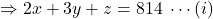As the number of two-point  goals was 49 less than double the number of three-point field goals she made.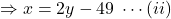Again, the number of free goals was 38 less than the number of two-point field goals she made.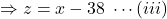From equations (i) and (iii)

2x+3y+x-38=814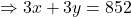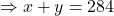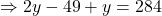[using equation (ii)]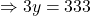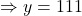From equation (ii),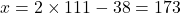From equation (iii),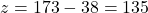.

Hence,

The number of free throws, z= 135

The number of two-point throw, x=173

The number of three-point throw, y=111.